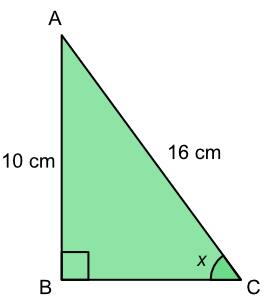Cos

# Cos

GCSE(F), GCSE(H),

The ratio of the adjacent side to the hypotenuse is the cosine, or cos of the angle. The adjacent side is the non-hypotenuse side that touches the angle.If an angle and one length (hypotenuse or adjacent) are known, find the cos value for that angle: substitute this value into the formula along with the known length.

If the two lengths (hypotenuse and adjacent) are known, the formula gives the cosine of the angle. The value of the angle is obtained by using the inverse operation (cos-1).

## Examples

1. What is the length of BC? Give your answer to 2 decimal places.cos x = frac(text(adjacent))(text(hypotenuse)

cos 36 = frac(text(BC))(12)

0.8090 x 12 = BC = 9.708 = 9.71 (2dp)

2. What is the value of the angle x? Give your answer to 1 decimal place.Answer: 38.7º

cos x = frac(text(opposite))(text(hypotenuse)

cos x = frac(10)(16)

cos x = 0.625

x = sin-1 0.625

x = 38.68º = 38.7º (1dp)